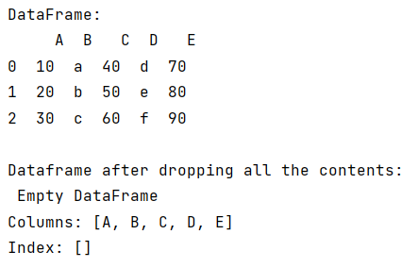# Python - Drop all data in a pandas dataframe

Given a Pandas DataFrame, we need to drop the entire contents of this DataFrame.
Submitted by Pranit Sharma, on July 28, 2022

Pandas is a special tool that allows us to perform complex manipulations of data effectively and efficiently. Inside pandas, we mostly deal with a dataset in the form of DataFrame. DataFrames are 2-dimensional data structures in pandas. DataFrames consist of rows, columns, and data.

To drop all the data in a DataFrame, pandas has a method called pandas.DataFrame.drop() method. It allows us to remove the column according to the column name.

## pandas.DataFrame.drop() Method

This method is used to remove a specified row or column from the pandas DataFrame. Since rows and columns are based on index and axis values respectively, by passing the index or axis value inside pandas.DataFrame.drop() method we can delete that particular row or column. Below is the syntax,

```df.drop(axis=None) # Deletes a specified column
```

To drop all the rows, we will have to pass all the indexes inside pandas.DataFrame.drop(), by using df.index, we can pass the entire row and column structure in this method.

Let us understand with the help of an example,

## Python code to drop all data in a pandas dataframe

```# Importing pandas package
import pandas as pd

# Creating a dictionary
d = {
'A':[10,20,30],
'B':['a','b','c'],
'C':[40,50,60],
'D':['d','e','f'],
'E':[70,80,90]
}

# Creating a dataframe
df = pd.DataFrame(d)

# Display Dataframe
print("DataFrame:\n",df,"\n")

# Droping the Dataframe
df.drop(df.index , inplace=True)

# Display modified DataFrame
print("Dataframe after dropping all the contents:\n",df)
```

Output:Languages: » C » C++ » C++ STL » Java » Data Structure » C#.Net » Android » Kotlin » SQL
Web Technologies: » PHP » Python » JavaScript » CSS » Ajax » Node.js » Web programming/HTML
Solved programs: » C » C++ » DS » Java » C#
Aptitude que. & ans.: » C » C++ » Java » DBMS
Interview que. & ans.: » C » Embedded C » Java » SEO » HR
CS Subjects: » CS Basics » O.S. » Networks » DBMS » Embedded Systems » Cloud Computing
» Machine learning » CS Organizations » Linux » DOS
More: » Articles » Puzzles » News/Updates